# Alluvial Diagrams in ggplot2

#### 2018-02-03

The ggalluvial package strives to adapt the style and flexibility of the alluvial package to the principles and frameworks of the tidyverse. This vignette

• defines the essential components of alluvial diagrams as used in the naming schemes and documentation (axis, alluvium, stratum, lode, flow),
• describes the alluvial data structures recognized by ggalluvial,
• illustrates the new stats and geoms, and
• showcases some popular variants on the theme and how to produce them.

Many other resources exist for visualizing categorical data in R, including several more basic plot types that are likely to more accurately convey proportions to viewers when the data are not so structured as to warrant an alluvial diagram. In particular, check out Michael Friendly’s vcd and vcdExtra packages (PDF) for a variety of statistically-motivated categorical data visualization techniques, Hadley Wickham’s productplots package and Haley Jeppson and Heike Hofmann’s descendant ggmosaic package for product or mosaic plots, and Nicholas Hamilton’s ggtern package for ternary coordinates. Other related packages are mentioned below.

## Alluvial diagrams

Here’s a quintessential alluvial diagram: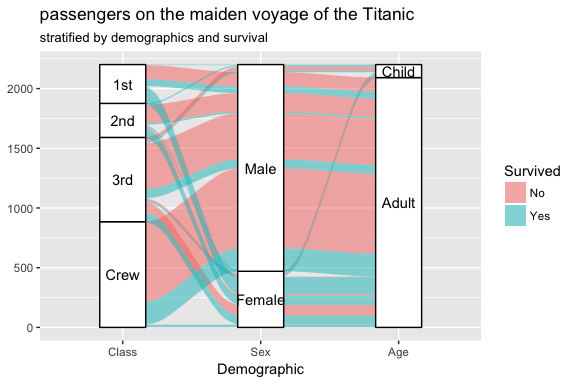The next section details how the elements of this image encode information about the underlying dataset. For now, we use the image as a point of reference to define the following elements of a typical alluvial diagram:

• An axis is a dimension (variable) along which the data are vertically grouped at a fixed horizontal position. The diagram above uses three categorical axes: Class, Sex, and Age.
• The groups at each axis are depicted as opaque blocks called strata. For example, the Class axis contains four strata: 1st, 2nd, 3rd, and Crew.
• Horizontal (x-) splines called alluvia span the width of the diagram. In this diagram, each alluvium corresponds to a fixed value of each axis variable, indicated by its vertical position at the axis, as well as of the Survived variable, indicated by its fill color.
• The segments of the alluvia between pairs of adjacent axes are flows.
• The alluvia intersect the strata at lodes. The lodes are not visualized in the above diagram, but they can be inferred as filled rectangles extending the flows through the strata at each end of the diagram or connecting the flows on either side of the center stratum.

As the examples in the next section will demonstrate, which of these elements are incorporated into an alluvial diagram depends on both how the underlying data is structured and what the creator wants the diagram to communicate.

## Alluvial data

ggalluvial recognizes two formats of “alluvial data”, treated in detail in the following subsections, but which basically correspond to the “wide” and “long” formats of categorical repeated measures data. A third, tabular (or array), form is popular for storing data with multiple categorical dimensions, such as the Titanic and UCBAdmissions datasets.1 For consistency with tidy data principles and ggplot2 conventions, ggalluvial does not accept tabular input; base::data.frame() converts such an array to an acceptable data frame.

### Alluvia (wide) format

The wide format reflects the visual arrangement of an alluvial diagram, but “untwisted”: Each row corresponds to a cohort of observations that take a specific value at each variable, and each variable has its own column. An additional column contains the weight of each row, e.g. the number of observational units in the cohort. This is the format into which the base function as.data.frame() transforms a frequency table, for instance the 3-dimensional UCBAdmissions dataset:

head(as.data.frame(UCBAdmissions), n = 12)
##       Admit Gender Dept Freq
## 1  Admitted   Male    A  512
## 2  Rejected   Male    A  313
## 3  Admitted Female    A   89
## 4  Rejected Female    A   19
## 5  Admitted   Male    B  353
## 6  Rejected   Male    B  207
## 7  Admitted Female    B   17
## 8  Rejected Female    B    8
## 9  Admitted   Male    C  120
## 10 Rejected   Male    C  205
## 11 Admitted Female    C  202
## 12 Rejected Female    C  391
is_alluvial(as.data.frame(UCBAdmissions), logical = FALSE, silent = TRUE)
##  "alluvia"

This format is inherited from the first version of ggalluvial, which modeled it after usage in alluvial: The user declares any number of axis variables, which stat_alluvium() and stat_stratum() recognize and process in a consistent way:

ggplot(as.data.frame(UCBAdmissions),
aes(weight = Freq, axis1 = Gender, axis2 = Dept)) +
geom_alluvium(aes(fill = Admit), width = 1/12) +
geom_stratum(width = 1/12, fill = "black", color = "grey") +
geom_label(stat = "stratum", label.strata = TRUE) +
scale_x_continuous(breaks = 1:2, labels = c("Gender", "Dept")) +
scale_fill_brewer(type = "qual", palette = "Set1") +
ggtitle("UC Berkeley admissions and rejections, by sex and department")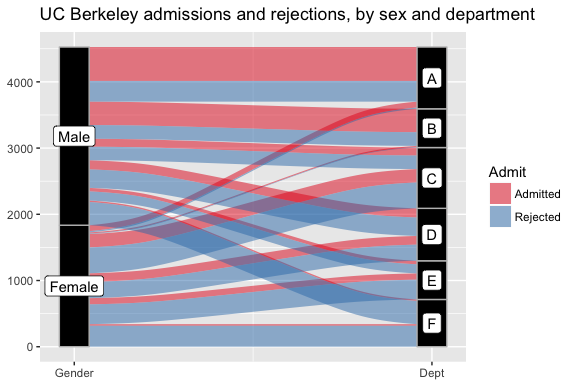An important feature of these diagrams is the meaningfulness of the vertical axis: No gaps are inserted between the strata, so the total height of the diagram reflects the cumulative weight of the observations. The diagrams produced by ggalluvial conform (somewhat; keep reading) to the “grammar of graphics” principles of ggplot2, and this prevents users from producing “free-floating” diagrams like the Sankey diagrams showcased here.2 ggalluvial parameters and existing ggplot2 functionality can also produce parallel sets plots, illustrated here using the Titanic dataset:3

ggplot(as.data.frame(Titanic),
aes(weight = Freq,
axis1 = Survived, axis2 = Sex, axis3 = Class)) +
geom_alluvium(aes(fill = Class),
width = 0, knot.pos = 0, reverse = FALSE) +
guides(fill = FALSE) +
geom_stratum(width = 1/8, reverse = FALSE) +
geom_text(stat = "stratum", label.strata = TRUE, reverse = FALSE) +
scale_x_continuous(breaks = 1:3, labels = c("Survived", "Sex", "Class")) +
coord_flip() +
ggtitle("Titanic survival by class and sex")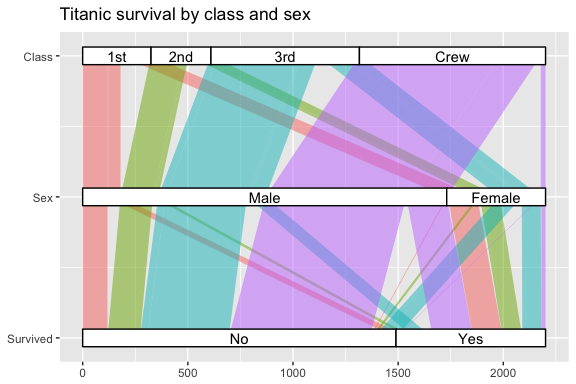This format and functionality are useful for many applications and will be retained in future versions. They also involve some conspicuous deviations from ggplot2 norms:

• The axis[0-9]* position aesthetics are non-standard: they are not an explicit set of parameters but a family based on a regular expression pattern; and at least one, but no specific one, is required.
• stat_alluvium() ignores any argument to the group aesthetic; instead, StatAlluvium$compute_panel() uses group to link the rows of the internally-transformed dataset that correspond to the same alluvium. • The label.strata parameter instructs stat_stratum() (called by geom_text()) to take the values of the axis variables as labels. • The horizontal axis must be manually corrected (using scale_x_continuous()) to reflect the implicit categorical variable identifying the axis. Furthermore, format aesthetics like fill are necessarily fixed for each alluvium; they cannot, for example, change from axis to axis according to the value taken at each. This means that, although they can reproduce the branching-tree structure of parallel sets, this format and functionality cannot produce alluvial diagrams with the color schemes featured here (“Alluvial diagram”) and here (“Controlling colors”), which are “reset” at each axis. ### Lodes (long) format The long format recognized by ggalluvial contains one row per lode, and can be understood as the result of “gathering” (in the dplyr sense) or “pivoting” (in the Microsoft Excel sense) the axis columns of a dataset in the alluvia format into a key-value pair of columns encoding the axis as the key and the stratum as the value. This format requires an additional indexing column that links the rows corresponding to a common cohort, i.e. the lodes of a single alluvium, as illustrated below using the to_lodes() defaults on the Berkeley admissions dataset: UCB_lodes <- to_lodes(as.data.frame(UCBAdmissions), axes = 1:3) head(UCB_lodes, n = 12) ## Freq alluvium x stratum ## 1 512 1 Admit Admitted ## 2 313 2 Admit Rejected ## 3 89 3 Admit Admitted ## 4 19 4 Admit Rejected ## 5 353 5 Admit Admitted ## 6 207 6 Admit Rejected ## 7 17 7 Admit Admitted ## 8 8 8 Admit Rejected ## 9 120 9 Admit Admitted ## 10 205 10 Admit Rejected ## 11 202 11 Admit Admitted ## 12 391 12 Admit Rejected is_alluvial(UCB_lodes, logical = FALSE, silent = TRUE) ##  "alluvia" The functions that convert data between wide (alluvia) and long (lodes) format include several parameters that help preserve ancillary information. See help("alluvial-data") for examples. The same stat and geom can receive data in this format using a different set of positional aesthetics, also specific to ggalluvial: • x, the “key” variable indicating the axis to which the row corresponds, which are to be arranged along the horizontal axis; • stratum, the “value” taken by the axis variable indicated by x; and • alluvium, the indexing scheme that links the rows of a single alluvium. Weights (and weight totals) can vary from axis to axis, allowing users to produce bump charts like those showcased here.4 In these cases, the strata are an artifact of the alluvia and often not plotted. For convenience, both stat_alluvium() and stat_flow() will accept arguments for x and alluvium even if none is given for stratum.5 As an example, we can group countries in the Refugees dataset by region, in order to compare refugee volumes at different scales: data(Refugees, package = "alluvial") country_regions <- c( Afghanistan = "Middle East", Burundi = "Central Africa", Congo DRC = "Central Africa", Iraq = "Middle East", Myanmar = "Southeast Asia", Palestine = "Middle East", Somalia = "Horn of Africa", Sudan = "Central Africa", Syria = "Middle East", Vietnam = "Southeast Asia" ) Refugees$region <- country_regions[Refugees$country] ggplot(data = Refugees, aes(x = year, weight = refugees, alluvium = country)) + geom_alluvium(aes(fill = country, colour = country), alpha = .75, decreasing = FALSE) + scale_x_continuous(breaks = seq(2003, 2013, 2)) + theme(axis.text.x = element_text(angle = -30, hjust = 0)) + scale_fill_brewer(type = "qual", palette = "Set3") + scale_color_brewer(type = "qual", palette = "Set3") + facet_wrap(~ region, scales = "fixed") + ggtitle("refugee volume by country and region of origin") ## Warning in f(...): Some color or differentiation aesthetics vary within ## alluvia; values at the first axis will be diffused across each alluvium.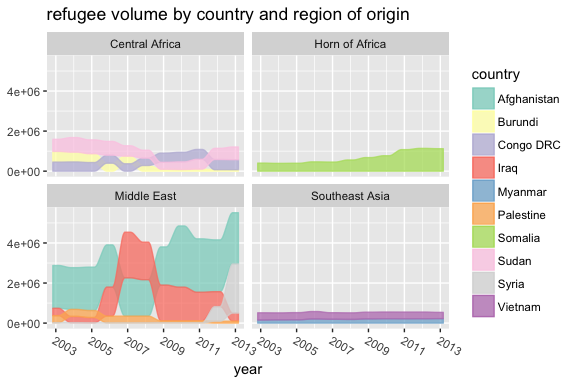The format allows us to assign aesthetics that change from axis to axis along the same alluvium, which is useful for repeated measures datasets. This requires generating a separate graphical object for each flow, as implemented in geom_flow(). The plot below uses a set of (changes to) students’ academic curricula over the course of several semesters. Since geom_flow() calls stat_flow() by default (see the next example), we override it with stat_alluvium() in order to track each student across all semesters: data(majors) majors$curriculum <- as.factor(majors$curriculum) ggplot(majors, aes(x = semester, stratum = curriculum, alluvium = student, fill = curriculum, label = curriculum)) + scale_fill_brewer(type = "qual", palette = "Set2") + geom_flow(stat = "alluvium", lode.guidance = "rightleft", color = "darkgray") + geom_stratum() + theme(legend.position = "bottom") + ggtitle("student curricula across several semesters")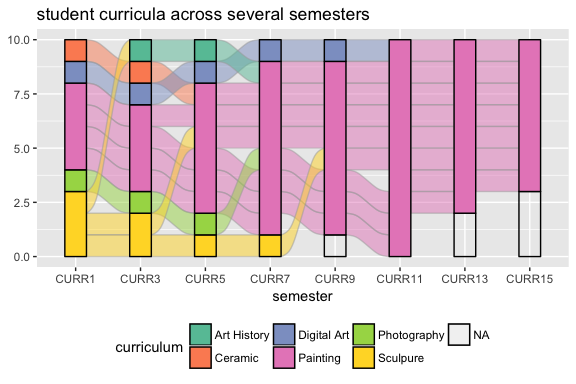No weight is specified, so each row is assigned unit weight. This example demonstrates one way ggalluvial handles missing data. The alternative is to set the parameter na.rm to TRUE.6 Missing data handling (specifically, the order of the strata) also depends on whether the stratum variable is character or factor/numeric. Finally, lode format gives us the option to aggregate the flows between adjacent axes, which may be appropriate when the transitions between adjacent axes are of primary importance. We can demonstrate this option on data from the influenza vaccination surveys conducted by the RAND American Life Panel: data(vaccinations) levels(vaccinations$response) <- rev(levels(vaccinations\$response))
ggplot(vaccinations,
aes(x = survey, stratum = response, alluvium = subject,
weight = freq,
fill = response, label = response)) +
geom_flow() +
geom_stratum(alpha = .5) +
geom_text(stat = "stratum", size = 3) +
theme(legend.position = "none") +
ggtitle("vaccination survey responses at three points in time")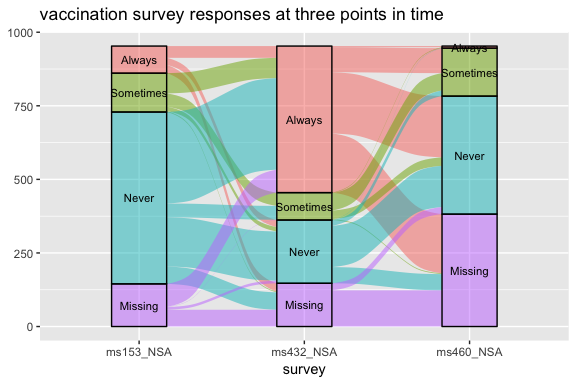This diagram ignores any continuity between the flows between axes. This “memoryless” plot produces a less cluttered diagram, in which at most one flow proceeds from each stratum at one axis to each stratum at the next, but at the cost of being able to track each cohort across the entire diagram.

## Appendix

Michał Bojanowski makes a habit of including R session info in each vignette. This makes eminent sense to me, so i’m doing it here.

sessionInfo()
## R version 3.3.2 (2016-10-31)
## Platform: x86_64-apple-darwin13.4.0 (64-bit)
## Running under: OS X Mavericks 10.9.5
##
## locale:
##  C/en_US.UTF-8/en_US.UTF-8/C/en_US.UTF-8/en_US.UTF-8
##
## attached base packages:
##  stats     graphics  grDevices utils     datasets  methods   base
##
## other attached packages:
##  bindrcpp_0.2       ggalluvial_0.6.0   ggplot2_2.2.1.9000
##
## loaded via a namespace (and not attached):
##   Rcpp_0.12.15       bindr_0.1          knitr_1.18
##   magrittr_1.5       tidyselect_0.2.3   munsell_0.4.3
##   colorspace_1.3-2   R6_2.2.2           rlang_0.1.6.9003
##  stringr_1.2.0      plyr_1.8.4         dplyr_0.7.4
##  tools_3.3.2        grid_3.3.2         gtable_0.2.0
##  withr_2.1.1.9000   htmltools_0.3.6    assertthat_0.2.0
##  yaml_2.1.16        lazyeval_0.2.1     rprojroot_1.3-2
##  digest_0.6.14      tibble_1.4.2       RColorBrewer_1.1-2
##  purrr_0.2.4        tidyr_0.7.2        glue_1.2.0
##  evaluate_0.10.1    rmarkdown_1.8      labeling_0.3
##  stringi_1.1.6      pillar_1.1.0       scales_0.5.0.9000
##  backports_1.1.2    pkgconfig_2.0.1

1. See Friendly’s tutorial, linked above, for a discussion.

2. The ggforce package includes parallel set geom and stat layers to produce similar diagrams that can be allowed to free-float.

3. A greater variety of parallel sets plots are implemented in the ggparallel package.

4. If bumping is unnecessary, consider using geom_area() instead.

5. stat_stratum() will similarly accept arguments for x and stratum without alluvium. If both strata and either alluvia or flows are to be plotted, though, all three parameters need arguments.

6. Be sure to set na.rm consistently in each layer, in this case both the flows and the strata.